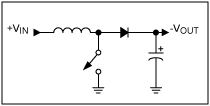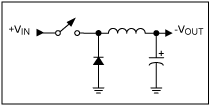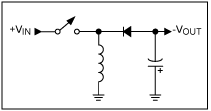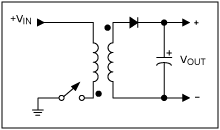# DC-DC Circuit Calculator

## An inductor, which stores energy, limits the current slew rate through a power switch. The energy stored in the inductor can be expressed in joules as a function of the current with: E = 1/2 X L X I2

Inputs
Input Voltage
V
Output Voltage
V
Output Current
mA
Output Voltage Ripple Factor
mv (pp)
Operating Frequency
kHz

Output
Timing Capacitor (Ct)
Rated Peak Withstand Current (Ipk)
Limited to Flow Resistance (Rsc)
Inductance (Lmin)
Filter Capacitor (Co)
Resistor 1 (R1)
Resistor 2 (R2)

### Equations & TopologyFigure 1. Simple boost converterFigure 2. Buck converter topologyFigure 3. Inverting topology.Figure 4. Transformer flyback topology.

The average voltage of an inductor over the switching cycle is zero in a steady-state operating condition. With this, when calculating for the boost circuit:

$$VIN X tON = tOFF X VL$$

And because:

$$VOUT = VIN + VL$$

We can then establish the relationship:

$$VOUT = VIN × (1 + tON/tOFF)$$

Using the relationship for duty cycle (D):

$$tON/(tON + tOFF) = D$$

Then for the boost circuit:

$$VOUT = VIN/(1-D)$$

Similar derivations can be made for the buck circuit:

$$VOUT = VIN × D$$

And for the inverter circuit (flyback):

$$VOUT = VIN × D/(1-D)$$

### Application

Switching power supplies are more efficient than linear power supplies.

1 Comment• B
Bharati July 07, 2022

INPUT VOLTAGE 50-85V DC
INPUT CURRENT 2.5A
OUTPUT VOLTAGE(NO LOAD CONDITION) 13.5 ± 0.5V
OUTPUT CURRENT 7.5A